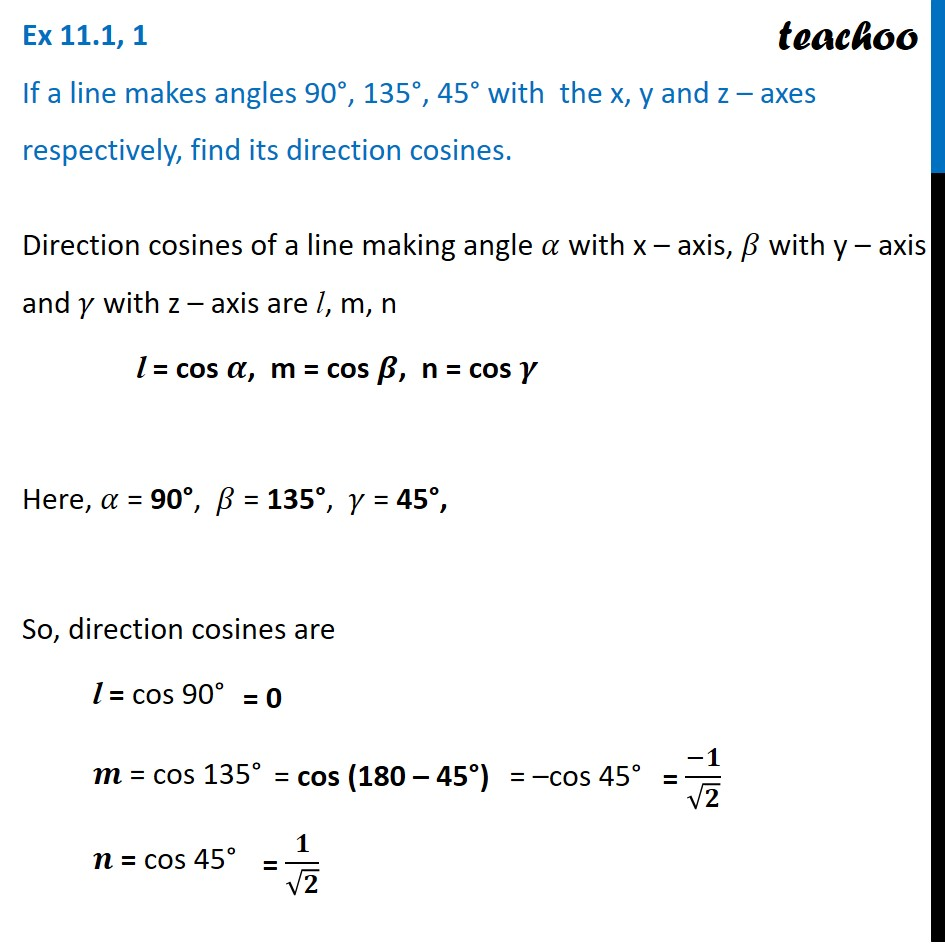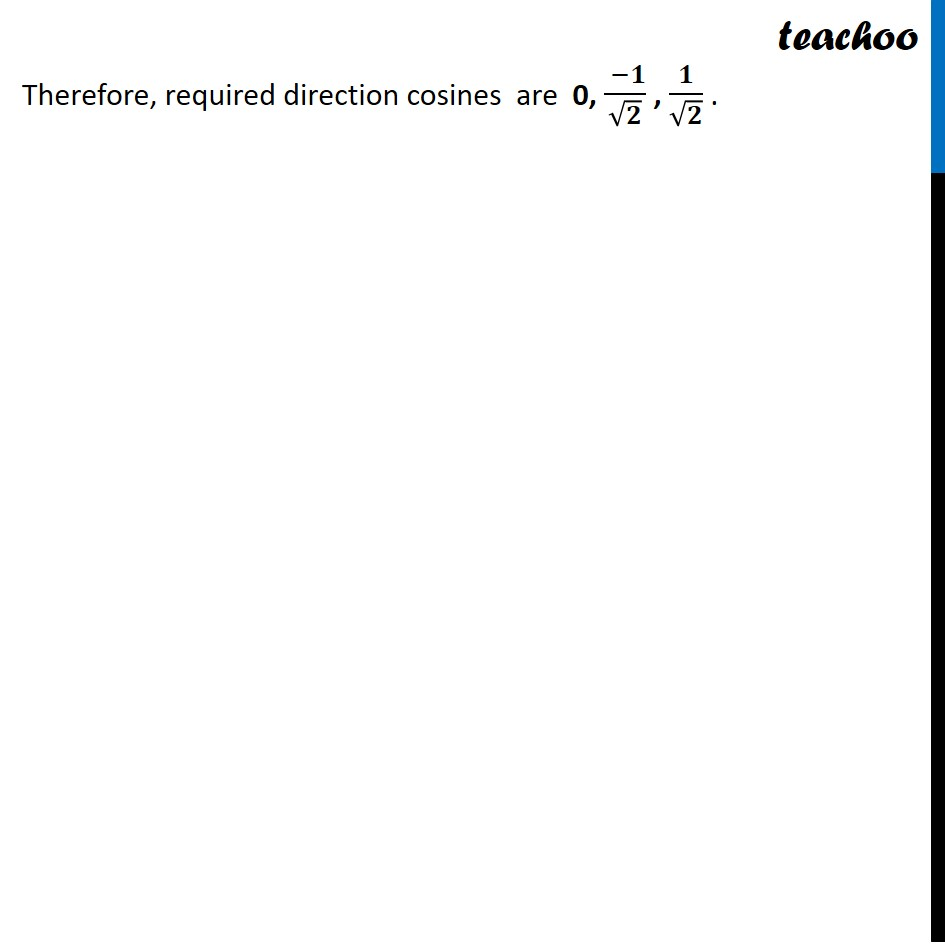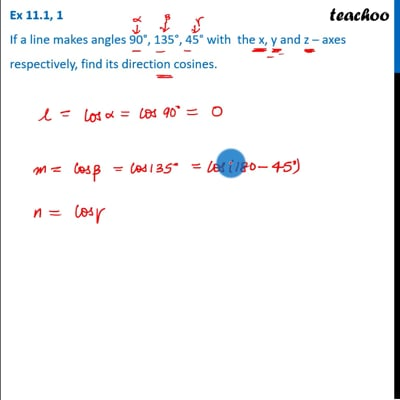Ex 11.1

Chapter 11 Class 12 Three Dimensional Geometry
Serial order wiseThis video is only available for Teachoo black users

Solve all your doubts with Teachoo Black (new monthly pack available now!)

### Transcript

Ex 11.1, 1 If a line makes angles 90°, 135°, 45° with the x, y and z – axes respectively, find its direction cosines. Direction cosines of a line making angle 𝛼 with x – axis, 𝛽 with y – axis and 𝛾 with z – axis are l, m, n l = cos 𝜶, m = cos 𝜷, n = cos 𝜸 Here, 𝛼 = 90°, 𝛽 = 135°, 𝛾 = 45°, So, direction cosines are l = cos 90° 𝒎 = cos 135° 𝒏 = cos 45° = 0 = cos (180 – 45°) = –cos 45° = (−𝟏)/√𝟐 = 𝟏/√𝟐 Therefore, required direction cosines are 0, ( −𝟏)/√𝟐 , 𝟏/√𝟐 .